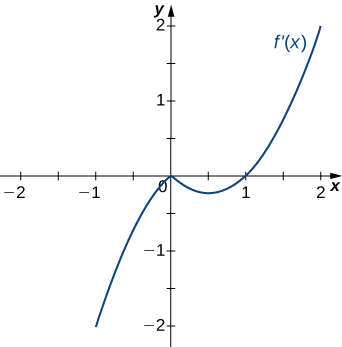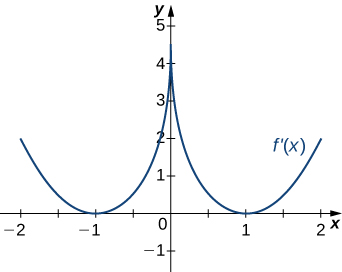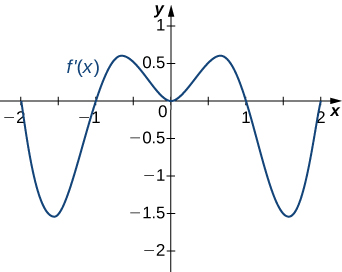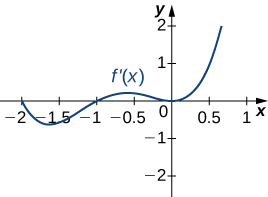$$\newcommand{\id}{\mathrm{id}}$$ $$\newcommand{\Span}{\mathrm{span}}$$ $$\newcommand{\kernel}{\mathrm{null}\,}$$ $$\newcommand{\range}{\mathrm{range}\,}$$ $$\newcommand{\RealPart}{\mathrm{Re}}$$ $$\newcommand{\ImaginaryPart}{\mathrm{Im}}$$ $$\newcommand{\Argument}{\mathrm{Arg}}$$ $$\newcommand{\norm}{\| #1 \|}$$ $$\newcommand{\inner}{\langle #1, #2 \rangle}$$ $$\newcommand{\Span}{\mathrm{span}}$$

4.3E: Shape of the Graph Exercises

$$\newcommand{\vecs}{\overset { \rightharpoonup} {\mathbf{#1}} }$$ $$\newcommand{\vecd}{\overset{-\!-\!\rightharpoonup}{\vphantom{a}\smash {#1}}}$$$$\newcommand{\id}{\mathrm{id}}$$ $$\newcommand{\Span}{\mathrm{span}}$$ $$\newcommand{\kernel}{\mathrm{null}\,}$$ $$\newcommand{\range}{\mathrm{range}\,}$$ $$\newcommand{\RealPart}{\mathrm{Re}}$$ $$\newcommand{\ImaginaryPart}{\mathrm{Im}}$$ $$\newcommand{\Argument}{\mathrm{Arg}}$$ $$\newcommand{\norm}{\| #1 \|}$$ $$\newcommand{\inner}{\langle #1, #2 \rangle}$$ $$\newcommand{\Span}{\mathrm{span}}$$ $$\newcommand{\id}{\mathrm{id}}$$ $$\newcommand{\Span}{\mathrm{span}}$$ $$\newcommand{\kernel}{\mathrm{null}\,}$$ $$\newcommand{\range}{\mathrm{range}\,}$$ $$\newcommand{\RealPart}{\mathrm{Re}}$$ $$\newcommand{\ImaginaryPart}{\mathrm{Im}}$$ $$\newcommand{\Argument}{\mathrm{Arg}}$$ $$\newcommand{\norm}{\| #1 \|}$$ $$\newcommand{\inner}{\langle #1, #2 \rangle}$$ $$\newcommand{\Span}{\mathrm{span}}$$

4.3: Derivatives and the Shape of a Graph

194) If c is a critical point of $$f(x)$$, when is there no local maximum or minimum at $$c$$? Explain.

195) For the function $$y=x^3$$, is $$x=0$$ both an inflection point and a local maximum/minimum?

It is not a local maximum/minimum because $$f′$$ does not change sign; it is an inflection point because $$f′′$$ does change sign.

196) For the function $$y=x^3$$, is $$x=0$$ an inflection point?

197) Is it possible for a point $$c$$ to be both an inflection point and a local extrema of a twice differentiable function?

No

198) Why do you need continuity for the first derivative test? Come up with an example.

199) Explain whether a concave-down function has to cross $$y=0$$ for some value of $$x$$.

False; for example, $$y=\sqrt{x}$$.

200) Explain whether a polynomial of degree $$2$$ can have an inflection point.

For the following exercises, analyze the graphs of $$f′$$, then list all intervals where f is increasing or decreasing.

201)Increasing for $$−2<x<−1$$ and $$x>2$$; decreasing for $$x<−2$$ and $$−1<x<2$$

202)203)Decreasing for $$x<1$$, increasing for $$x>1$$

204)205)Decreasing for $$−2<x<−1$$ and $$1<x<2$$; increasing for $$−1<x<1$$ and $$x<−2$$ and $$x>2$$

For the following exercises, analyze the graphs of $$f′,$$ then list all intervals where

a. $$f$$ is increasing and decreasing and

b. the minima and maxima are located.

206)207)a. Increasing over $$−2<x<−1,0<x<1,x>2$$, decreasing over $$x<−2, −1<x<0,1<x<2;$$
b. maxima at $$x=−1$$ and $$x=1$$, minima at $$x=−2$$ and $$x=0$$ and $$x=2$$

208)209)a. Increasing over $$x>0$$, decreasing over $$x<0;$$
b. Minimum at $$x=0$$

210)For the following exercises, analyze the graphs of $$f′$$, then list all inflection points and intervals $$f$$ that are concave up and concave down.

211)Concave up on all $$x$$, no inflection points

212)213)Concave up on all $$x$$, no inflection points (since f'(x) is always increasing)

214)215)Concave up for $$x<0$$ and $$x>1$$, concave down for $$0<x<1$$
inflection points at $$x=0$$ and $$x=1$$

For the following exercises, draw a graph that satisfies the given specifications for the domain $$x=[−3,3].$$ The function does not have to be continuous or differentiable.

216) $$f(x)>0,f′(x)>0$$ over $$x>1,−3<x<0,f′(x)=0$$ over $$0<x<1$$

217) $$f′(x)>0$$ over $$x>2,−3<x<−1,f′(x)<0$$ over $$−1<x<2,f''(x)<0$$ for all $$x$$

218) $$f''(x)<0$$ over $$−1<x<1,f''(x)>0,−3<x<−1,1<x<3,$$ local maximum at $$x=0,$$ local minima at $$x=±2$$

219) There is a local maximum at $$x=2,$$ local minimum at $$x=1,$$ and the graph is neither concave up nor concave down.

220) There are local maxima at $$x=±1,$$ the function is concave up for all $$x$$, and the function remains positive for all $$x.$$

For the following exercises, determine

a. intervals where $$f$$ is increasing or decreasing and

b. local minima and maxima of $$f$$.

221) $$f(x)=sinx+sin^3x$$ over −π<x<π

a. Increasing over $$−\frac{π}{2}<x<\frac{π}{2},$$ decreasing over $$x<−π\frac{π}{2},x>\frac{π}{2}$$

b. Local maximum at $$x=\frac{π}{2}$$; local minimum at $$x=−\frac{π}{2}$$

222) $$f(x)=x^2+cosx$$

For the following exercise, determine a. intervals where $$f$$ is concave up or concave down, and b. the inflection points of $$f$$.

223) $$f(x)=x^3−4x^2+x+2$$

a. Concave up for $$x>\frac{4}{3},$$ concave down for $$x<\frac{4}{3}$$

b. Inflection point at $$x=\frac{4}{3}$$

For the following exercises, determine

a. intervals where $$f$$ is increasing or decreasing,

b. local minima and maxima of $$f$$,

c. intervals where $$f$$ is concave up and concave down, and

d. the inflection points of $$f.$$

224) $$f(x)=x^2−6x$$

225) $$f(x)=x^3−6x^2$$

a. Increasing over $$x<0$$ and $$x>4,$$ decreasing over $$0<x<4$$
b. Maximum at $$x=0$$, minimum at $$x=4$$
c. Concave up for $$x>2$$, concave down for $$x<2$$
d. Infection point at $$x=2$$

226) $$f(x)=x^4−6x^3$$

227) $$f(x)=x^{11}−6x^{10}$$

a. Increasing over $$x<0$$ and $$x>\frac{60}{11}$$, decreasing over $$0<x<\frac{60}{11}$$ b. Minimum at $$x=\frac{60}{11}$$ c. Concave down for $$x<\frac{54}{11}$$, concave up for $$x>\frac{54}{11}$$ d. Inflection point at $$x=\frac{54}{11}$$

228) $$f(x)=x+x^2−x^3$$

229) $$f(x)=x^2+x+1$$

a. Increasing over $$x>−\frac{1}{2}$$, decreasing over $$x<−\frac{1}{2}$$ b. Minimum at $$x=−\frac{1}{2}$$ c. Concave up for all $$x$$ d. No inflection points

230) $$f(x)=x^3+x^4$$

For the following exercises, determine

a. intervals where $$f$$ is increasing or decreasing,

b. local minima and maxima of $$f,$$

c. intervals where $$f$$ is concave up and concave down, and

d. the inflection points of $$f.$$ Sketch the curve, then use a calculator to compare your answer. If you cannot determine the exact answer analytically, use a calculator.

231) [T] $$f(x)=sin(πx)−cos(πx)$$ over $$x=[−1,1]$$

a. Increases over $$−\frac{1}{4}<x<\frac{3}{4},$$ decreases over $$x>\frac{3}{4}$$ and $$x<−\frac{1}{4}$$ b. Minimum at $$x=−\frac{1}{4}$$, maximum at $$x=\frac{3}{4}$$ c. Concave up for $$−\frac{3}{4}<x<\frac{1}{4}$$, concave down for $$x<−\frac{3}{4}$$ and $$x>\frac{1}{4}$$ d. Inflection points at $$x=−\frac{3}{4},x=\frac{1}{4}$$

232) [T] $$f(x)=x+sin(2x)$$ over $$x=[−\frac{π}{2},\frac{π}{2}]$$

233) $$f(x)=sinx+tanx$$ over $$(−\frac{π}{2},\frac{π}{2})$$

a. Increasing for all $$x$$
b. No local minimum or maximum
c. Concave up for $$x>0$$, concave down for $$x<0$$
d. Inflection point at $$x=0$$

234) [T] $$f(x)=(x−2)^2(x−4)^2$$

235) [T] $$f(x)=\frac{1}{1−x},x≠1$$

a. Increasing for all $$x$$ where defined
b. No local minima or maxima
c. Concave up for $$x<1$$; concave down for $$x>1$$
d. No inflection points in domain

236) [T] $$f(x)=\frac{sinx}{x}$$ over $$x=[−2π,2π] [2π,0)∪(0,2π]$$

237) $$f(x)=sin(x)e^x$$ over $$x=[−π,π]$$

Solution: a. Increasing over $$−\frac{π}{4}<x<\frac{3π}{4}$$, decreasing over $$x>\frac{3π}{4},x<−\frac{π}{4}$$ b. Minimum at $$x=−\frac{π}{4}$$, maximum at $$x=\frac{3π}{4}$$ c. Concave up for $$−\frac{π}{2}<x<\frac{π}{2}$$, concave down for $$x<−\frac{π}{2},x>\frac{π}{2}$$ d. Infection points at $$x=±\frac{π}{2}$$

238) $$f(x)=lnx\sqrt{x},x>0$$

239) $$f(x)=\frac{1}{4}\sqrt{x}+\frac{1}{x},x>0$$

Solution: a. Increasing over $$x>4,$$ decreasing over $$0<x<4$$ b. Minimum at $$x=4$$ c. Concave up for $$0<x<8\sqrt{2}$$, concave down for $$x>8\sqrt{2}$$ d. Inflection point at $$x=8\sqrt{2}$$

240) $$f(x)=\frac{e^x}{x},x≠0$$

For the following exercises, interpret the sentences in terms of $$f,f′,$$ and $$f''.$$

241) The population is growing more slowly. Here $$f$$ is the population.

$$f>0,f′>0,f''<0$$

242) A bike accelerates faster, but a car goes faster. Here $$f=$$ Bike’s position minus Car’s position.

243) The airplane lands smoothly. Here $$f$$ is the plane’s altitude.

$$f>0,f′<0,f''<0$$

244) Stock prices are at their peak. Here $$f$$is the stock price.

245) The economy is picking up speed. Here $$f$$ is a measure of the economy, such as GDP.

$$f>0,f′>0,f''>0$$

For the following exercises, consider a third-degree polynomial $$f(x),$$ which has the properties f′(1)=0,f′(3)=0.

Determine whether the following statements are true or false. Justify your answer.

246) $$f(x)=0$$ for some $$1≤x≤3$$

247) $$f''(x)=0$$ for some $$1≤x≤3$$

248) There is no absolute maximum at $$x=3$$
249) If $$f(x)$$ has three roots, then it has $$1$$ inflection point.
250) If $$f(x)$$ has one inflection point, then it has three real roots.